Tutorials
python

Face Detection with Python using OpenCV

This tutorial will introduce you to the concept of object detection in Python using OpenCV library and how you can utilize it to perform tasks like Facial detection.

Introduction

Face detection is a computer vision technology that helps to locate/visualize human faces in digital images. This technique is a specific use case of object detection technology that deals with detecting instances of semantic objects of a certain class (such as humans, buildings or cars) in digital images and videos. With the advent of technology, face detection has gained a lot of importance especially in fields like photography, security, and marketing.

Pre-requisites

Hands-on knowledge of Numpy and Matplotlib is essential before working on the concepts of OpenCV. Make sure that you have the following packages installed and running before installing OpenCV.

1. OpenCV-Python

2. 1.Overview

3. 2.Installation

4. Images as Arrays

5. Binary Image

6. Grayscale Image
7. Colored Image

8. Images and OpenCV

9. Importing Images in OpenCV

10. Savings images
11. Basic Operations on Images

12. Face Detection

13. Overview

15. Face Detection with OpenCV-Python

1. OpenCV-Python

OverviewOpenCV was started at Intel in the year 1999 by Gary Bradsky. The first release came a little later in the year 2000. OpenCV essentially stands for Open Source Computer Vision Library. Although it is written in optimized C/C++, it has interfaces for Python and Java along with C++. OpenCV boasts of an active user base all over the world with its use increasing day by day due to the surge in computer vision applications.

OpenCV-Python is the python API for OpenCV. You can think of it as a python wrapper around the C++ implementation of OpenCV. OpenCV-Python is not only fast (since the background consists of code written in C/C++) but is also easy to code and deploy(due to the Python wrapper in foreground). This makes it a great choice to perform computationally intensive programs.

Installation

OpenCV-Python supports all the leading platforms like Mac OS, Linux, and Windows. It can be installed in either of the following ways:

1. From pre-built binaries and source :

Please refer to the detailed documentation here for Windows and here for Mac.

Packages for standard desktop environments (Windows, macOS, almost any GNU/Linux distribution)

• run pip install opencv-python if you need only main modules
• run pip install opencv-contrib-python if you need both main and contrib modules (check extra modules listing from OpenCV documentation)

You can either use Jupyter notebooks or any Python IDE of your choice for writing the scripts.

2. Images as Arrays

An image is nothing but a standard Numpy array containing pixels of data points. More the number of pixels in an image, the better is its resolution. You can think of pixels to be tiny blocks of information arranged in the form of a 2 D grid, and the depth of a pixel refers to the color information present in it. In order to be processed by a computer, an image needs to be converted into a binary form. The color of an image can be calculated as follows:

Number of colors/ shades = 2^bpp where bpp represents bits per pixel.

Naturally, more the number of bits/pixels, more possible colors in the images. The following table shows the relationship more clearly.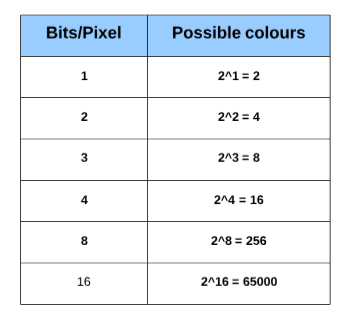1. Binary Image

A binary image consists of 1 bit/pixel and so can have only two possible colors, i.e., black or white. Black is represented by the value 0 while 1 represents white.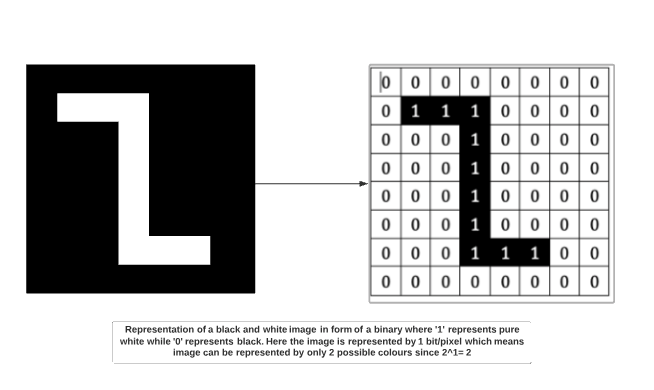2. Grayscale image

A grayscale image consists of 8 bits per pixel. This means it can have 256 different shades where 0 pixels will represent black color while 255 denotes white. For example, the image below shows a grayscale image represented in the form of an array. A grayscale image has only 1 channel where the channel represents dimension.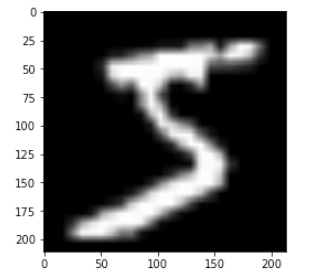3. Colored image

Colored images are represented as a combination of Red, Blue, and Green, and all the other colors can be achieved by mixing these primary colors in correct proportions.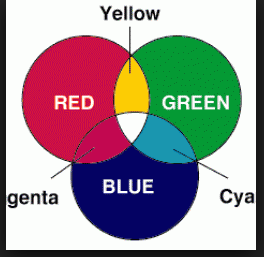source

A colored image also consists of 8 bits per pixel. As a result, 256 different shades of colors can be represented with 0 denoting black and 255 white. Let us look at the famous colored image of a mandrill which has been cited in many image processing examples.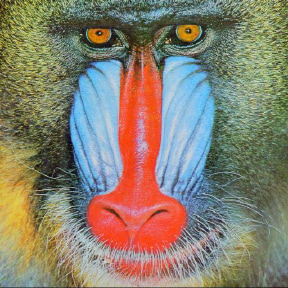If we were to check the shape of the image above, we would get:

Shape
(288, 288, 3)
288: Pixel width
288: Pixel height
3: color channel

This means we can represent the above image in the form of a three-dimensional array.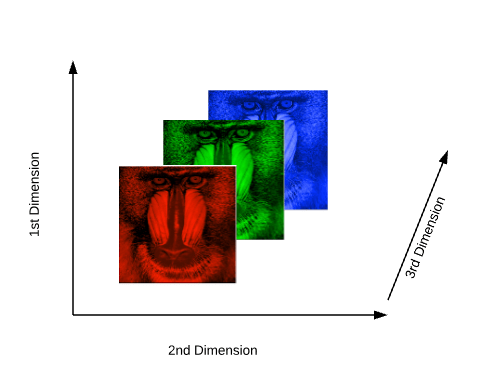3. Images and OpenCV

Before we jump into the process of face detection, let us learn some basics about working with OpenCV. In this section we will perform simple operations on images using OpenCV like opening images, drawing simple shapes on images and interacting with images through callbacks. This is necessary to create a foundation before we move towards the advanced stuff.

Importing Images in OpenCV

Using Jupyter notebooks

Steps:

• Import the necessary libraries
import numpy as np
import cv2
import matplotlib.pyplot as plt
%matplotlib inline
• Read in the image using the imread function. We will be using the colored 'mandrill' image for demonstration purpose. It can be downloaded from here
• The type and shape of the array.
type(img_raw)
numpy.ndarray

img_raw.shape
(1300, 1950, 3)

Thus, the .png image gets transformed into a numpy array with a shape of 1300x1950 and has 3 channels.

• viewing the image
plt.imshow(img_raw)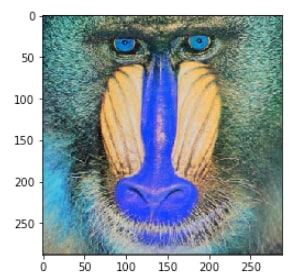What we get as an output is a bit different concerning color. We expected a bright colored image but what we obtain is an image with some bluish tinge. That happens because OpenCV and matplotlib have different orders of primary colors. Whereas OpenCV reads images in the form of BGR, matplotlib, on the other hand, follows the order of RGB. Thus, when we read a file through OpenCV, we read it as if it contains channels in the order of blue, green and red. However, when we display the image using matplotlib, the red and blue channel gets swapped and hence the blue tinge. To avoid this issue, we will transform the channel to how matplotlib expects it to be using the cvtColor function.

img = cv2.cvtColor(img_raw, cv2.COLOR_BGR2RGB)
plt.imshow(img_rgb)Using Python Scripts

Jupyter Notebooks are great for learning, but when dealing with complex images and videos, we need to display them in their own separate windows. In this section, we will be executing the code as a .py file. You can use Pycharm, Sublime or any IDE of your choice to run the script below.

import cv2
while True:
cv2.imshow('mandrill',img)

if cv2.waitKey(1) & 0xFF == 27:
break

cv2.destroyAllWindows()

In this code, we have a condition, and the image will only be shown if the condition is true. Also, to break the loop, we will have two conditions to fulfill:

• The cv2.waitKey() is a keyboard binding function. Its argument is the time in milliseconds. The function waits for specified milliseconds for any keyboard event. If you press any key in that time, the program continues.
• The second condition pertains to the pressing of the Escape key on the keyboard. Thus, if 1 millisecond has passed and the escape key is pressed, the loop will break and program stops.
• cv2.destroyAllWindows() simply destroys all the windows we created. If you want to destroy any specific window, use the function cv2.destroyWindow() where you pass the exact window name as the argument.

Savings images

The images can be saved in the working directory as follows:

cv2.imwrite('final_image.png',img)

Where the final_image is the name of the image to be saved.

Basic Operations on Images

In this section, we will learn how we can draw various shapes on an existing image to get a flavor of working with OpenCV.

Drawing on Images

• Begin by importing necessary libraries.
import numpy as np
import matplotlib.pyplot as plt
%matplotlib inline
import cv2
• Create a black image which will act as a template.
image_blank = np.zeros(shape=(512,512,3),dtype=np.int16)
• Display the black image.
plt.imshow(image_blank)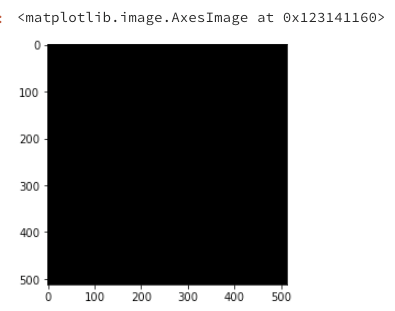Function & Attributes

The generalised function for drawing shapes on images is:

cv2.shape(line, rectangle etc)(image,Pt1,Pt2,color,thickness)

There are some common arguments which are passed in function to draw shapes on images:

• Image on which shapes are to be drawn
• co-ordinates of the shape to be drawn from Pt1(top left) to Pt2(bottom right)
• Color: The color of the shape that is to be drawn. It is passed as a tuple, eg: (255,0,0). For grayscale, it will be the scale of brightness.
• The thickness of the geometrical figure.

1. Straight Line

Drawing a straight line across an image requires specifying the points, through which the line will pass.

# Draw a diagonal red line with thickness of 5 px
line_red = cv2.line(img,(0,0),(511,511),(255,0,0),5)
plt.imshow(line_red)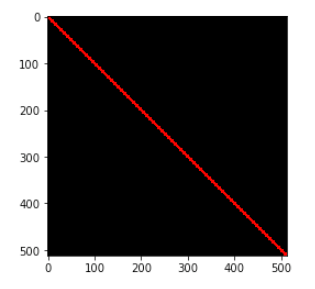# Draw a diagonal green line with thickness of 5 px
line_green = cv2.line(img,(0,0),(511,511),(0,255,0),5)
plt.imshow(line_green)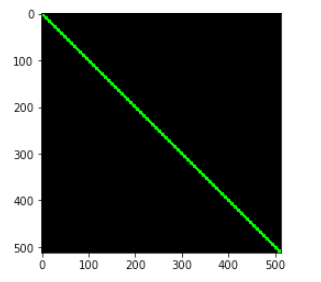2. Rectangle

For a rectangle, we need to specify the top left and the bottom right coordinates.

#Draw a blue rectangle with a thickness of 5 px

rectangle= cv2.rectangle(img,(384,0),(510,128),(0,0,255),5)
plt.imshow(rectangle)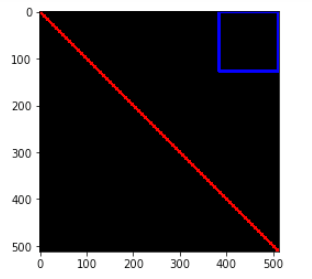3. Circle

For a circle, we need to pass its center coordinates and radius value. Let us draw a circle inside the rectangle drawn above

img = cv2.circle(img,(447,63), 63, (0,0,255), -1) # -1 corresponds to a filled circle
plt.imshow(circle)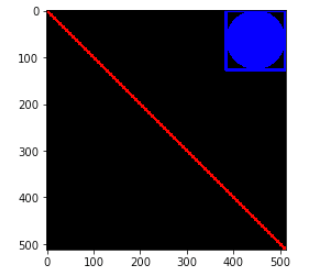Writing on Images

Adding text to images is also similar to drawing shapes on them. But you need to specify certain arguments before doing so:

• Text to be written
• coordinates of the text. The text on an image begins from the bottom left direction.
• Font type and scale.
• Other attributes like color, thickness and line type. Normally the line type that is used is lineType = cv2.LINE_AA.
font = cv2.FONT_HERSHEY_SIMPLEX
text = cv2.putText(img,'OpenCV',(10,500), font, 4,(255,255,255),2,cv2.LINE_AA)
plt.imshow(text)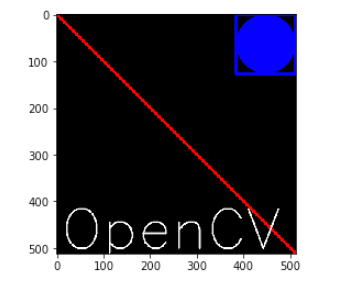These were the minor operations that can be done on images using OpenCV. Feel free to experiment with the shapes and text.

4.Face Detection

Overview

Face detection is a technique that identifies or locates human faces in digital images. A typical example of face detection occurs when we take photographs through our smartphones, and it instantly detects faces in the picture. Face detection is different from Face recognition. Face detection detects merely the presence of faces in an image while facial recognition involves identifying whose face it is. In this article, we shall only be dealing with the former.

Face detection is performed by using classifiers. A classifier is essentially an algorithm that decides whether a given image is positive(face) or negative(not a face). A classifier needs to be trained on thousands of images with and without faces. Fortunately, OpenCV already has two pre-trained face detection classifiers, which can readily be used in a program. The two classifiers are:

• Haar Classifier and
• Local Binary Pattern(LBP) classifier.

Haar-like features are digital image features used in object recognition. They owe their name to their intuitive similarity with Haar wavelets and were used in the first real-time face detector. Paul Viola and Michael Jones in their paper titled "Rapid Object Detection using a Boosted Cascade of Simple Features" used the idea of Haar-feature classifier based on the Haar wavelets. This classifier is widely used for tasks like face detection in computer vision industry.

Haar cascade classifier employs a machine learning approach for visual object detection which is capable of processing images extremely rapidly and achieving high detection rates. This can be attributed to three main reasons:

• Haar classifier employs 'Integral Image' concept which allows the features used by the detector to be computed very quickly.
• The learning algorithm is based on AdaBoost. It selects a small number of important features from a large set and gives highly efficient classifiers.
• More complex classifiers are combined to form a 'cascade' which discard any non-face regions in an image, thereby spending more computation on promising object-like regions.

Let us now try and understand how the algorithm works on images in steps:

1. 'Haar features' extraction

After the tremendous amount of training data (in the form of images) is fed into the system, the classifier begins by extracting Haar features from each image. Haar Features are kind of convolution kernels which primarily detect whether a suitable feature is present on an image or not. Some examples of Haar features are mentioned below: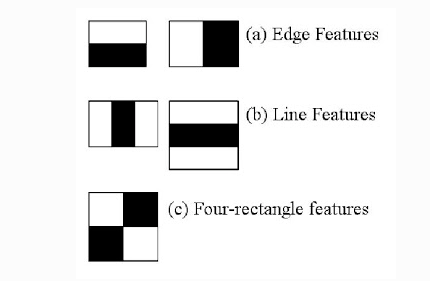source

These Haar Features are like windows and are placed upon images to compute a single feature. The feature is essentially a single value obtained by subtracting the sum of the pixels under the white region and that under the black. The process can be easily visualized in the example below.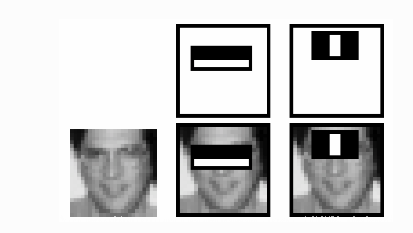For demonstration purpose, let's say we are only extracting two features, hence we have only two windows here. The first feature relies on the point that the eye region is darker than the adjacent cheeks and nose region. The second feature focuses on the fact that eyes are kind of darker as compared to the bridge of the nose. Thus, when the feature window moves over the eyes, it will calculate a single value. This value will then be compared to some threshold and if it passes that it will conclude that there is an edge here or some positive feature.

2. 'Integral Images' concept

The algorithm proposed by Viola Jones uses a 24X24 base window size, and that would result in more than 180,000 features being calculated in this window. Imagine calculating the pixel difference for all the features? The solution devised for this computationally intensive process is to go for the Integral Image concept. The integral image means that to find the sum of all pixels under any rectangle, we simply need the four corner values.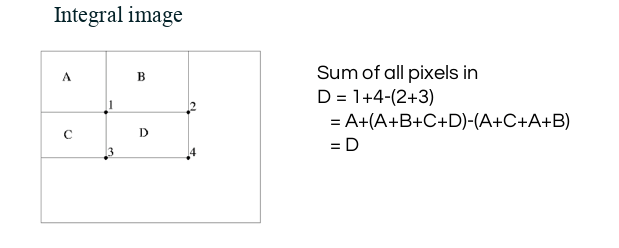source

This means, to calculate the sum of pixels in any feature window, we do not need to sum them up individually. All we need is to calculate the integral image using the 4 corner values. The example below will make the process transparent.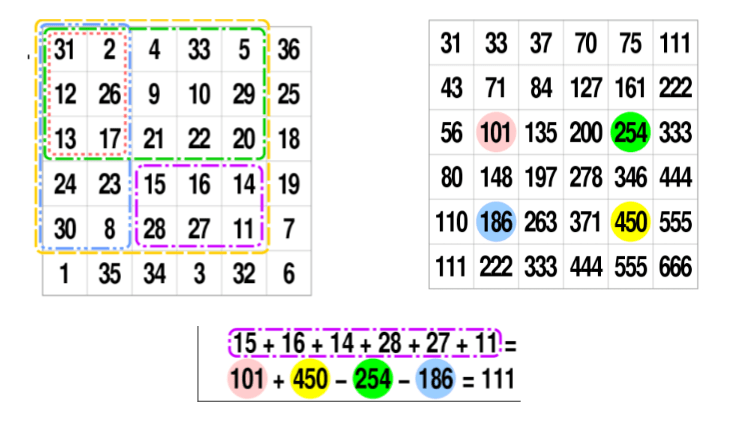source

3. 'Adaboost' : to improve classifier accuracy

As pointed out above, more than 180,000 features values result within a 24X24 window. However, not all features are useful for identifying a face. To only select the best feature out of the entire chunk, a machine learning algorithm called Adaboost is used. What it essentially does is that it selects only those features that help to improve the classifier accuracy. It does so by constructing a strong classifier which is a linear combination of a number of weak classifiers. This reduces the amount of features drastically to around 6000 from around 180,000.

Another way by which Viola Jones ensured that the algorithm performs fast is by employing a cascade of classifiers. The cascade classifier essentially consists of stages where each stage consists of a strong classifier. This is beneficial since it eliminates the need to apply all features at once on a window. Rather, it groups the features into separate sub-windows and the classifier at each stage determines whether or not the sub-window is a face. In case it is not, the sub-window is discarded along with the features in that window. If the sub-window moves past the classifier, it continues to the next stage where the second stage of features is applied. The process can be understood with the help of the diagram below.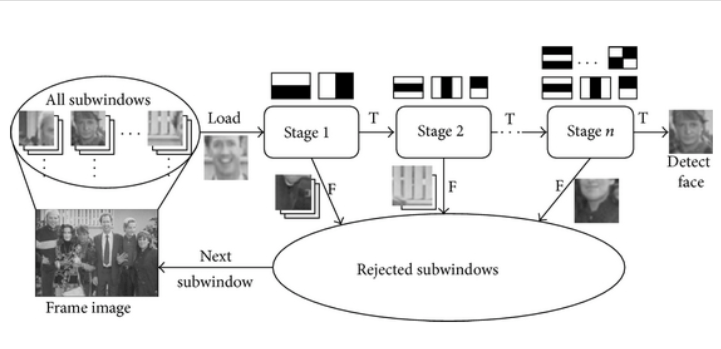Cascade structure for Haar classifiers.

The Paul- Viola algorithm can be visualized as follows: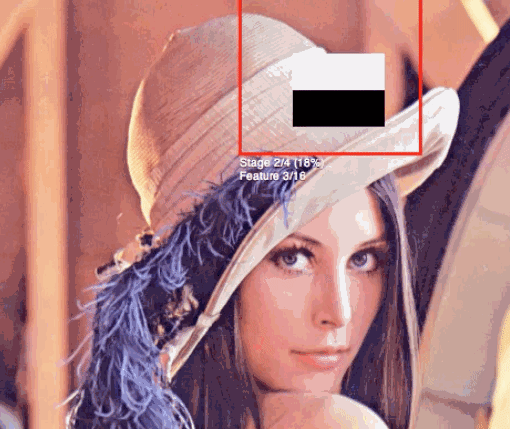source

Face Detection with OpenCV-Python

Now we have a fair idea about the intuition and the process behind Face recognition. Let us now use OpenCV library to detect faces in an image.

import numpy as np
import cv2
import matplotlib.pyplot as plt
%matplotlib inline

We shall be using the image below: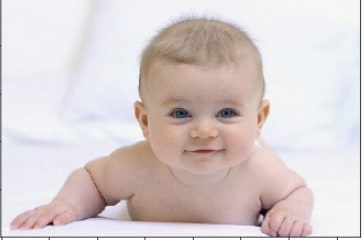#Converting to grayscale
test_image_gray = cv2.cvtColor(test_image, cv2.COLOR_BGR2GRAY)

# Displaying the grayscale image
plt.imshow(test_image_gray, cmap='gray')
Since we know that OpenCV loads an image in BGR format, so we need to convert it into RBG format to be able to display its true colors. Let us write a small function for that.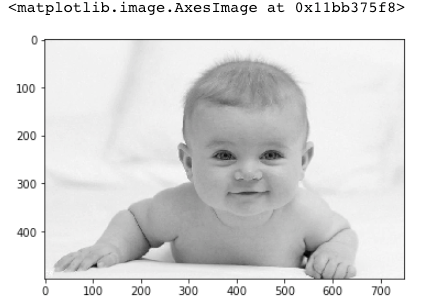Since we know that OpenCV loads an image in BGR format, so we need to convert it into RBG format to be able to display its true colors. Let us write a small function for that.

def convertToRGB(image):
return cv2.cvtColor(image, cv2.COLOR_BGR2RGB)

OpenCV comes with a lot of pre-trained classifiers. For instance, there are classifiers for smile, eyes, face, etc. These come in the form of xml files and are located in the opencv/data/haarcascades/ folder. However, to make things simple, you can also access them from here. Download the xml files and place them in the data folder in the same working directory as the jupyter notebook.

Face detection

We shall be using the detectMultiscale module of the classifier. This function will return a rectangle with coordinates(x,y,w,h) around the detected face. This function has two important parameters which have to be tuned according to the data.

• scalefactor In a group photo, there may be some faces which are near the camera than others. Naturally, such faces would appear more prominent than the ones behind. This factor compensates for that.

• minNeighbors This parameter specifies the number of neighbors a rectangle should have to be called a face. You can read more about it here.

faces_rects = haar_cascade_face.detectMultiScale(test_image_gray, scaleFactor = 1.2, minNeighbors = 5);

# Let us print the no. of faces found
print('Faces found: ', len(faces_rects))

Faces found:  1

Our next step is to loop over all the coordinates it returned and draw rectangles around them using Open CV. We will be drawing a green rectangle with a thickness of 2

for (x,y,w,h) in faces_rects:
cv2.rectangle(test_image, (x, y), (x+w, y+h), (0, 255, 0), 2)

Finally, we shall display the original image in colored to see if the face has been detected correctly or not.

#convert image to RGB and show image

plt.imshow(convertToRGB(test_image))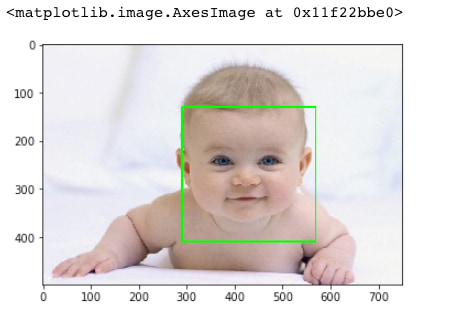Here, it is. We have successfully detected the face of the baby in the picture. Let us now create a generalized function for the entire face detection process.

Face Detection with generalized function

def detect_faces(cascade, test_image, scaleFactor = 1.1):
# create a copy of the image to prevent any changes to the original one.
image_copy = test_image.copy()

#convert the test image to gray scale as opencv face detector expects gray images
gray_image = cv2.cvtColor(image_copy, cv2.COLOR_BGR2GRAY)

# Applying the haar classifier to detect faces

for (x, y, w, h) in faces_rect:
cv2.rectangle(image_copy, (x, y), (x+w, y+h), (0, 255, 0), 15)

return image_copy

Testing the function on new image

This time test image is as follows: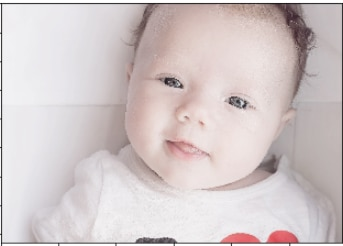# Converting to grayscale

test_image_gray = cv2.cvtColor(test_image, cv2.COLOR_BGR2GRAY)

# Displaying grayscale image

plt.imshow(test_image_gray, cmap='gray')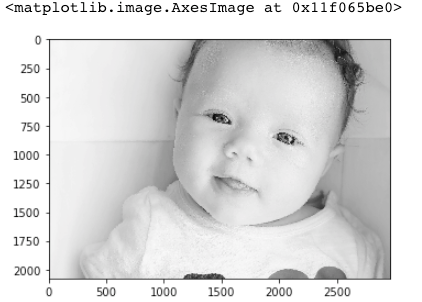#call the function to detect faces

#convert to RGB and display image
plt.imshow(convertToRGB(faces))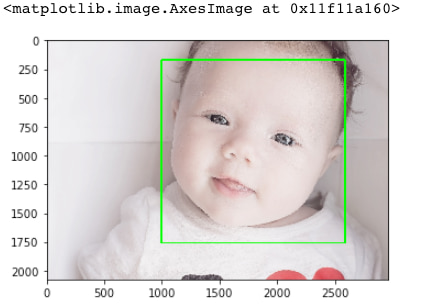Testing the function on a group image

Let us now see if the function works well on a group photograph or not. We shall be using the picture below for our purpose.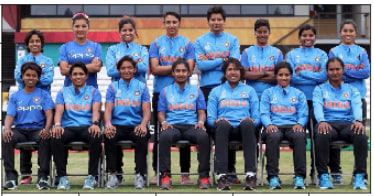Image: The Indian Women's Cricket Team.

#call the function to detect faces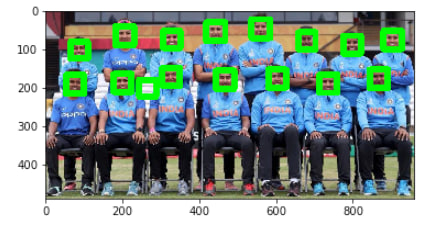In this tutorial, we learned about the concept of face detection using Open CV in Python using Haar cascade. There are a number of detectors other than the face, which can be found in the library. Feel free to experiment with them and create detectors for eyes, license plates, etc. For any queries, please leave a comment below. `